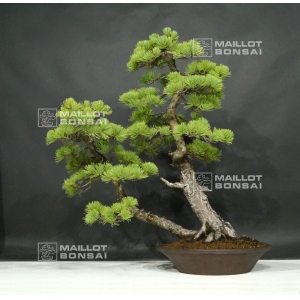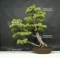##### The Japanese Bonsai specialist
Direct order Contact Help / Services Newsletter# Pinus pentaphylla 13080182

Pinus pentaphyllaref. : 100401.880,00

voluminous/heavy item extra shipping of 12,00

Available quantity : 1Order

###### Description

Height of the tree without its pot : 850 mm. Width of the branches : 700 mm. Japanese non-enamelled pottery of : 470 x 110 mm. Sokan style with 2 trunks. Diameter of each trunk's base : 50/80 mm. Nebari (root's base) of about 240 mm. Bonsai aged of more than 40-45 years old. Beautiful ramifications without very strong cutting wounds or ligature wire marks. Well-conical trunk with its formed bark that presents a wonderful movement. This tree currently has ligature wire to lower his branches, give it much more character to this superb bonsaï.

Presence of jins on its trunk.

Photographed in August 2018. Comes from Takahashi's japanese nursery.

#bonsai 4.6 #trunk 3.5 #pentaphylla 3.1 #coniferous 3 #japanese 2.8 #ligature 2.8 #branches 2.8 #without 2.7 #pinus 2.5 #wire 2.4

Formule
(( ROUND((CHAR_LENGTH(b.article_nom)-CHAR_LENGTH(REPLACE(b.article_nom, 'trunk', '')))/LENGTH('trunk')) + ROUND((CHAR_LENGTH(b.article_description)-CHAR_LENGTH(REPLACE(b.article_description, 'trunk', '')))/LENGTH('trunk')) ) * 3.5) + (( ROUND((CHAR_LENGTH(b.article_nom)-CHAR_LENGTH(REPLACE(b.article_nom, 'pentaphylla', '')))/LENGTH('pentaphylla')) + ROUND((CHAR_LENGTH(b.article_description)-CHAR_LENGTH(REPLACE(b.article_description, 'pentaphylla', '')))/LENGTH('pentaphylla')) ) * 3.1) + (( ROUND((CHAR_LENGTH(b.article_nom)-CHAR_LENGTH(REPLACE(b.article_nom, 'branches', '')))/LENGTH('branches')) + ROUND((CHAR_LENGTH(b.article_description)-CHAR_LENGTH(REPLACE(b.article_description, 'branches', '')))/LENGTH('branches')) ) * 2.8) + (( ROUND((CHAR_LENGTH(b.article_nom)-CHAR_LENGTH(REPLACE(b.article_nom, 'ligature', '')))/LENGTH('ligature')) + ROUND((CHAR_LENGTH(b.article_description)-CHAR_LENGTH(REPLACE(b.article_description, 'ligature', '')))/LENGTH('ligature')) ) * 2.8) + (( ROUND((CHAR_LENGTH(b.article_nom)-CHAR_LENGTH(REPLACE(b.article_nom, 'japanese', '')))/LENGTH('japanese')) + ROUND((CHAR_LENGTH(b.article_description)-CHAR_LENGTH(REPLACE(b.article_description, 'japanese', '')))/LENGTH('japanese')) ) * 2.8) + (( ROUND((CHAR_LENGTH(b.article_nom)-CHAR_LENGTH(REPLACE(b.article_nom, 'without', '')))/LENGTH('without')) + ROUND((CHAR_LENGTH(b.article_description)-CHAR_LENGTH(REPLACE(b.article_description, 'without', '')))/LENGTH('without')) ) * 2.7) + (( ROUND((CHAR_LENGTH(b.article_nom)-CHAR_LENGTH(REPLACE(b.article_nom, 'bonsai', '')))/LENGTH('bonsai')) + ROUND((CHAR_LENGTH(b.article_description)-CHAR_LENGTH(REPLACE(b.article_description, 'bonsai', '')))/LENGTH('bonsai')) ) * 2.6) + (( ROUND((CHAR_LENGTH(b.article_nom)-CHAR_LENGTH(REPLACE(b.article_nom, 'pinus', '')))/LENGTH('pinus')) + ROUND((CHAR_LENGTH(b.article_description)-CHAR_LENGTH(REPLACE(b.article_description, 'pinus', '')))/LENGTH('pinus')) ) * 2.5) + (( ROUND((CHAR_LENGTH(b.article_nom)-CHAR_LENGTH(REPLACE(b.article_nom, 'this', '')))/LENGTH('this')) + ROUND((CHAR_LENGTH(b.article_description)-CHAR_LENGTH(REPLACE(b.article_description, 'this', '')))/LENGTH('this')) ) * 2.4) + (( ROUND((CHAR_LENGTH(b.article_nom)-CHAR_LENGTH(REPLACE(b.article_nom, 'with', '')))/LENGTH('with')) + ROUND((CHAR_LENGTH(b.article_description)-CHAR_LENGTH(REPLACE(b.article_description, 'with', '')))/LENGTH('with')) ) * 2.4)

## Secure payment## Delivery

Our logistic partners :04 74 55 23 48
Pépinière MAILLOT-BONSAÏ
Le Bois Frazy
01990 RELEVANT - FRANCE
on appointment